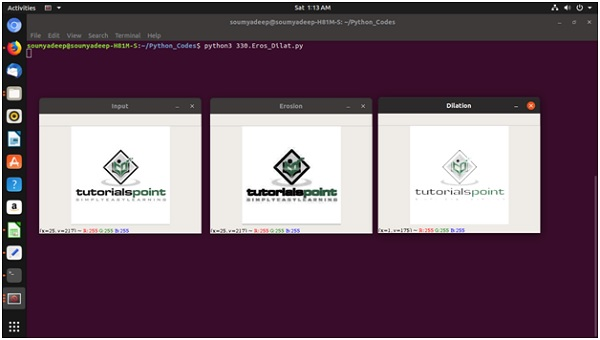# Erosion and Dilation of images using OpenCV in Python

In this problem, we will see how Python can do some Morphological Operations like Erosion and Dilation using the OpenCV module. The OpenCV library is mainly designed for computer vision. It is open source. Originally it was designed by Intel. This is free to use under open-source BSD license.

To use the OpenCV functionality, we need to download them using pip.

sudo pip3 install opencv-python


## What is Erosion Image and how it works?

In the Erosion, it erodes away the boundaries of foreground objects. It is used to remove small white noises from the images. Erosion can also be used to detach two connected images.

• A kernel is formed from an image. The kernel is a matrix, where the order is odd, like 3, 5, 7.

• A pixel of image is chosen. This pixel will be chosen as 1 only if all the pixels under the kernel is 1. Otherwise it will be eroded.

• So all pixels near the boundary will be discarded.

• So the thickness of foreground object decreases.

## What is Dilation Image and how it works?

In the Dilation, it increases the object area. The Erosion can remove the white noises, but it also shrinks our image, so after Erosion, if Dilation is performed, we can get better noise removal results. The Dilation can also be used to joins some broken parts of an object.

• A kernel is formed from an image. The kernel is a matrix, where the order is odd, like 3, 5, 7.

• A pixel of image is chosen. This pixel will be chosen as 1 only if all the pixels under the kernel is 1.

• Increases the white region or the size of foreground objects of the image.

## Example Code

import cv2
import numpy as np
kernel = np.ones((3,3), np.uint8)       # set kernel as 3x3 matrix from numpy
#Create erosion and dilation image from the original image
erosion_image = cv2.erode(input_image, kernel, iterations=1)
dilation_image = cv2.dilate(input_image, kernel, iterations=1)
cv2.imshow('Input', input_image)
cv2.imshow('Erosion', erosion_image)
cv2.imshow('Dilation', dilation_image)
cv2.waitKey(0)       #wait for a key to exit


## Output